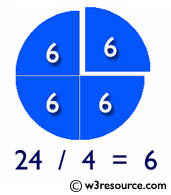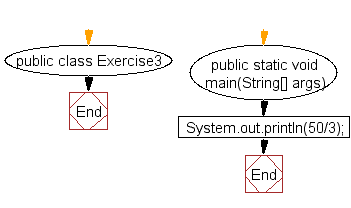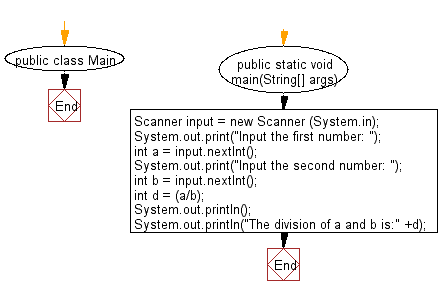﻿ Java exercises: Divide two numbers and print on the screen - w3resource# Java Exercises: Divide two numbers and print on the screen

## Java Basic: Exercise-3 with Solution

Write a Java program to divide two numbers and print on the screen.

Division is one of the four basic operations of arithmetic, the others being addition, subtraction, and multiplication. The division of two natural numbers is the process of calculating the number of times one number is contained within one another.

Pictorial Presentation:Sample Solution:

Java Code:

``````public class Exercise3 {

public static void main(String[] args) {
System.out.println(50/3);
}

}
```
```

Sample Output:

```16
```

Flowchart:Sample solution using input from the user:

Java Code:

``````import java.util.Scanner;

public class Main {
public static void main(String[] args)
{
Scanner input = new Scanner (System.in);
System.out.print("Input the first number: ");
int a = input.nextInt();
System.out.print("Input the second number: ");
int b = input.nextInt();
int d = (a/b);
System.out.println();
System.out.println("The division of a and b is:" +d);
}
}``````

Sample Output:

```Input the first number:  7
Input the second number:  2

The division of a and b is:3
```

Flowchart:Java Code Editor:

What is the difficulty level of this exercise?

Test your Programming skills with w3resource's quiz.

﻿

## Java: Tips of the Day

countOccurrences

Counts the occurrences of a value in an array.

Use Arrays.stream().filter().count() to count total number of values that equals the specified value.

```public static long countOccurrences(int[] numbers, int value) {
return Arrays.stream(numbers)
.filter(number -> number == value)
.count();
}
```

Ref: https://bit.ly/3kCAgLb